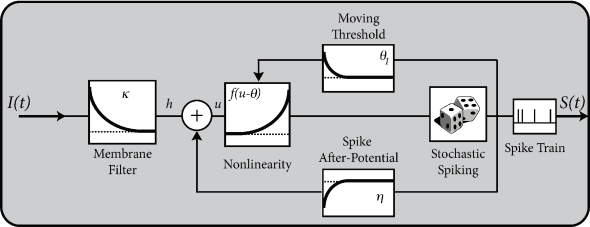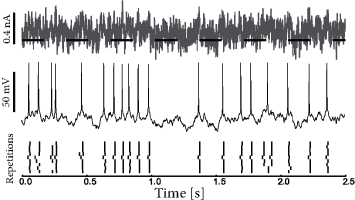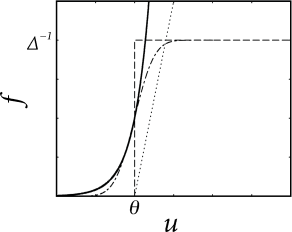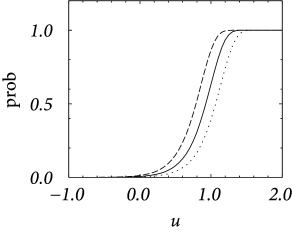# 9.1 Escape noise

In the escape noise model, we imagine that an integrate-and-fire neuron with threshold $\vartheta$ can fire even though the formal threshold $\vartheta$ has not been reached or may stay quiescent even though the formal threshold has transiently been passed. To do this consistently, we introduce an ‘escape rate’ or ‘firing intensity’ which depends on the momentary state of the neuron.

# 9.1.1 Escape rate

Given the input $I(t^{\prime})$ for $t^{\prime} and the past firing times $t^{(f)}, the membrane potential of a generalized integrate-and-fire model (e.g., the adaptive leaky ingrate-and-fire model) or a Spike Response Model (SRM) can be calculated from Eq. (6.7) or Eq. (6.27) respectively; cf. Chapter 6. For example, the value of the membrane potential of a SRM can be expressed as

 $\displaystyle u(t)=\sum_{f}\eta(t-t^{(f)})+\int_{0}^{\infty}\kappa(s)\,I^{\rm det% }(t-s)\,{\text{d}}s+u_{\rm rest},$ (9.1)

where $I^{\rm det}$ is the known driving current (the superscript ‘det’ stands for deterministic) and $\kappa$ and $\eta$ are filters that describe the response of the membrane to an incoming pulse or an outgoing spike; see Chapter 6. In the deterministic model the next spike occurs when $u$ reaches the threshold $\vartheta$. In order to introduce some variability into the neuronal spike generator, we replace the strict threshold by a stochastic firing criterion. In the noisy threshold model, spikes can occur at any time with a probability density

 $\rho(t)=f(u(t)-\vartheta)\,,$ (9.2)

that depends on the momentary distance between the (noiseless) membrane potential and the threshold; see Fig. 9.1. We can think of $f$ as an escape rate similar to the one encountered in models of chemical reactions (529). In the mathematical theory of point processes, the quantity $\rho$ is called a ‘stochastic intensity’. Since we use $\rho$ in the context of neuron models we will refer to it as a firing intensity.Fig. 9.1: Noisy threshold. A neuron can fire at time tt with probability density ρ⁢(t)=f⁢[u⁢(t)-ϑ]\rho(t)=f[u(t)-\vartheta] even though the membrane potential uu has not yet reached the threshold ϑ\vartheta. In other words, the sharp threshold of the deterministic neuron is replaced by a ‘soft’ threshold.Fig. 9.2: Flow diagram for a Spike Response Model (SRM) with escape noise. The stochasticity at the noisy threshold is indicated by the dice; cf. Fig. 6.11. The noisy SRM (180; 177) is an example of a Generalized Linear Model (GLM) (521; 399).

The choice of the escape function $f$ in Eq. (9.2) is arbitrary. A reasonable condition is to require $f\to 0$ for $u\to-\infty$ so that the neuron does not fire if the membrane potential is far below threshold. A common choice is the exponential,

 $f(u-\vartheta)={1\over\tau_{0}}\,\exp[\beta\,(u-\vartheta)]\,,$ (9.3)

where $\beta$ and $\tau_{0}$ are parameters. For $\beta\to\infty$, the soft threshold turns into a sharp one so that we return to the noiseless model. Below we discuss some further choices of the escape function in Eq. (9.2).

The SRM of Eq. (9.1) together with the exponential escape rate of Eq. (9.3) is an example of a Generalized Linear Model. Applying the theory of Generalized Linear Models (cf. Chapter 10) to the SRM with escape noise enables a rapid extraction of model parameters from experimental data.

In slice experiments it was found (247) that the exponential escape rate of Eq. (9.3) provides an excellent fit to the spiking intensity of real neurons (Fig. 9.3). Moreover, the firing times of an AdEx model driven by a deterministic fluctuating current $I^{\rm det}(t)$ and a unknown white noise current $\xi(t)$ can also be well fitted by the Spike Response Model of Eq. (9.1) combined with an exponential escape rate as we will see below in Section 9.4.

Nevertheless, we may wonder whether Eq. (9.2) is a sufficiently general noise model. We have seen in Chapter 2 that the concept of a pure voltage threshold is questionable. More generally, the spike trigger process could, for example, also depend on the slope $\dot{u}=du/dt$ with which the ‘threshold’ is approached. In the noisy threshold model, we may therefore also consider an escape rate (or hazard) which depends not only on $u$ but also on its derivative $\dot{u}$

 $\rho(t)=f[u(t)-\vartheta,\dot{u}(t)]\,.$ (9.4)Fig. 9.3: The instantaneous firing intensity extracted from experiments can be fitted by an exponential escape rate. A. A real neuron is driven by a time-dependent input current (top) generating a fluctuating voltage with occasional spikes (middle), which are repeated with high precision, but not perfectly, across several trials (bottom). B. The black histogram (very small) shows the number of times (bin count, vertical axis) that the model voltage calculated from Eq. (9.1) falls in the bin u-ϑu-\vartheta (horizontal axis) and the real neuron fires. Gray histogram indicates distribution of voltage when the real neuron does not fire. The ratio (black/black plus gray) in each bin gives the firing probability PF⁢(u-ϑ)P_{F}(u-\vartheta) (open circles, probability scale on the right) which can be fitted by Eq. (9.8) using an exponential escape rate (solid line), f⁢(u-ϑ)=1τ0⁢exp⁡[β⁢(u-ϑ)]f(u-\vartheta)={1\over\tau_{0}}\,\exp[\beta\,(u-\vartheta)] with a steepness of β=(4⁢mV)-1\beta=(4\,{\rm mV})^{-1} and a mean latency at threshold of τ0=19\tau_{0}=19 ms. From Jolivet et al. 2006.

Note that the time-dependence of the firing intensity $\rho(t)$ on the right-hand side of Eq. (9.4) arises implicitly via the membrane potential $u(t)$. In an even more general model, we could in addition include an explicit time dependence, e.g., to account for a reduced spiking probability immediately after a spike at $t^{(f)}$. Instead of an explicit dependence, a slightly more convenient way to implement an additional time dependence is via a time-dependent threshold $\vartheta\longrightarrow\vartheta(t)$ which we have already encountered in Eq. (6.31). An even more general escape rate model therefore is

 $\rho(t)=f[u(t)-\vartheta(t),\dot{u}(t)]\,.$ (9.5)

In Chapter 11 we will see that a Spike Response Model with escape noise and dynamic threshold can explain neuronal firing with a high degree of accuracy.

Example: Bounded versus unbounded escape rate

A stochastic intensity which diverges for $u\gg\vartheta$ such as the exponential escape rate of Eq. (9.3) may seem surprising at a first glance, but it is in fact a necessary requirement for the transition from a soft to a sharp threshold process. Since the escape model has been introduced as a noisy threshold, there should be a limit of low noise that leads us back to the sharp threshold. In order to explore the relation between noisy and deterministic threshold models, we consider as a first step a bounded escape function $f$ defined as

 $f(u-\vartheta)=\left\{\begin{array}[]{*{2}{c@{\qquad}}c}0\qquad&{\rm for}% \qquad&u<\vartheta\\ \Delta^{-1}\qquad&{\rm for}\qquad&u\geq\vartheta\end{array}\right.$ (9.6)

Thus, the neuron never fires if the voltage is below the threshold. If $u>\vartheta$, the neuron fires stochastically with a rate $\Delta^{-1}$. Therefore, the mean latency, or expected delay, of a spike is $\Delta$. This implies that the neuron responds slowly and imprecisely, even when the membrane potential is significantly above threshold – and this result looks rather odd. Only in the limit where the parameter $\Delta$ goes to zero, the neuron would fire immediately and reliably as soon as the membrane potential crosses the threshold. Thus, a rapid response requires the escape to diverge.

The argument here was based on a step function for the escape rate. A simple choice for a soft threshold which enables a rapid response is a piecewise linear escape rate,

 $f(u-\vartheta)=\alpha\,[u-\vartheta]_{+}=\left\{\begin{array}[]{*{2}{c@{\qquad% }}c}0\qquad&{\rm for}\qquad&u<\vartheta\\ \alpha\,(u-\vartheta)\qquad&{\rm for}\qquad&u\geq\vartheta\end{array}\right.$ (9.7)

with slope $\alpha$ for $u>\vartheta$. For $u>\vartheta$, the firing intensity is proportional to $u-\vartheta$; cf. Fig. 9.4. This corresponds to the intuitive idea that instantaneous firing rates increase with the membrane potential. Variants of the linear escape-rate model are commonly used to describe spike generation in, e.g., auditory nerve fibers (475; 347).Fig. 9.4: Soft threshold escape rates. Exponential function (solid), piecewise linear function (dotted), step function (dashed), and error function (dot-dashed). The step function and error function saturate at a maximum rate of Δ-1\Delta^{-1}. The threshold is ϑ\vartheta.

# 9.1.2 Transition from continuous time to discrete time

In discrete time, we consider the probability $P_{F}(u)$ of firing in a finite time step given the neurons has membrane potential $u$. It is bounded from above by one – despite the fact that the escape rate can be arbitrarily large. We start from a model in continuous time and discretize time as it is often done in simulations. In a straightforward discretization scheme, we would calculate the probability of firing during a time step $\Delta t$ as $\int_{t}^{t+\Delta t}\rho(t^{\prime}){\text{d}}t^{\prime}\approx\rho(t)\,\Delta t$. However, for $u\gg\vartheta$, the hazard $\rho(t)=f[u(t)-\vartheta]$ can take large values; see, e.g., Eq. (9.3). Thus $\Delta t$ must be taken extremely short so as to guarantee $\rho(t)\,\Delta t<1$.

In order to arrive at an improved discretization scheme, we calculate the probability that a neuron does not fire in a time step $\Delta t$. The probability $S(t)$ to survive for a time $t$ without firing decays according to $dS/dt=-\rho(t)\,S(t)$. Integration of the differential equation over a finite time $\Delta t$ yields an exponential factor $S(t)=\exp[-\int_{0}^{t}\rho(t^{\prime})\,{\text{d}}t^{\prime}]$; compare the discussion of the survivor function in Chapter 7 (Section 7.5). If the neuron does not survive, it must have fired. Therefore we arrive at a firing probability

 $P_{F}(u)={\rm Prob}\left\{{\rm spike~{}in~{}}[t,t+\Delta t]\,|\,u(t)\right\}% \approx 1-\exp\left\{-\Delta t\,f[u(t)-\vartheta]\right\}\,.$ (9.8)

Even if $f$ diverges for $u\to\infty$, the probability of firing remains bounded between zero and one. Aslo, we see that for small $\Delta t$, the probability $P_{F}$ scales as $f\Delta t$. We see from Fig. 9.5A that, for an exponential escape rate, an increase in the discretization $\Delta t$ mainly shifts the firing curve to the left while the form remains roughly the same. An increase of the noise level makes the curve flatter; cf. Fig. 9.5B.

Note that, because of refractoriness, it is impossible for integrate-and-fire models (and for real neurons) to fire more than one spike in a short time bin $\Delta t$. Refractoriness is implemented in the model of Eq. (9.1) by a significant reset of the membrane potential via the refractory kernel $\eta$.Fig. 9.5: The unbounded exponential escape rate yields a bounded firing probability in a discrete time step. A. Probability of firing in a discrete time interval Δ⁢t\Delta t as a function of the membrane potential uu for different discretizations Δ⁢t=0.5\Delta t=0.5  ms (dashed line), Δ⁢t=1\Delta t=1 ms (solid line), and Δ⁢t=2\Delta t=2 ms (dotted line) with β=5\beta=5. B. Similar plot as in A but for different noise levels β=10\beta=10 (dotted line), β=5\beta=5 (solid line), β=2\beta=2 (dashed line), and β=1\beta=1 (dot-dashed line) with Δ⁢t=1\Delta t=1  ms. The escape rate is given by (9.3) with parameters ϑ=1\vartheta=1 and τ0=1\tau_{0}=1\,ms.